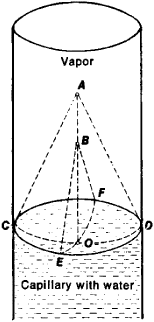# Laplace's Law

Also found in: Medical, Wikipedia.

## Laplace’s Law

the dependence of the hydrostatic pressure drop Δp at the boundary surface between two phases (liquid-liquid, liquid-gas, or liquid-vapor) on the interphase surface tension σ and the mean curvature ∊ of the surface at a given point: Δp = P1P2 = ∊σ, where p1 is the pressure on the concave side of the surface, p2 is the pressure on the convex side, and ∊ = 1/R1 + 1/R2 where R1 and R2 are the radii of curvature in two mutually perpendicular normal sections of the surface at the given point (see Figure 1).Figure 1. Example of application of Laplace’s law to water vapor interface in a capillary: Δp = p1p2. R1, and R2, the radii of curvature at point 0 of the concave surface (R1, = OA and R2 = OB), are determined in two mutually perpendicular sections ACD and BEF.

The Laplace law, established in 1806 by P. Laplace, determines the magnitude of the capillary pressure, and thereby makes it possible to write down the conditions of mechanical equilibrium for movable (liquid) interfaces.

Mentioned in ?
References in periodicals archive ?
If such conformational changes are found to occur during spaceflight, then this could provide a driving mechanism for the observed cardiac remodeling that is based upon simple physical forces as described by Laplace's Law.
However, in accordance with Laplace's law, the geometry of the heart and its impact on the segmental wall stress should act as a driving force for cardiac remodeling.
Laplace's law dictates that the intraluminal pressure needed to stretch the wall of a hollow tube is inversely proportional to its radius.

Site: Follow: Share:
Open / Close